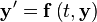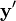# ODE

NLA
QRSlv
CMSlv
IPSlv
NLP
CONOPT
IPOPT
TRON
MINOS
Opt
NGSlv
DAE/ODE
IDA
LSODE
DOPRI5
LA
Linsolqr
Linsol
LP
MakeMPS
Logic
LRSlv

A system of ordinary differential equations (an ODE system) can be defined$\bold{y}' = \bold{f} \left(t,\bold{y} \right)$

where$\bold{y}$ is a vector of variables and$\bold{y}'$ is the corresponding vector of derivatives with respect to the 'time' or 'independent' variable$t$. Written in the above form, we can always be sure that we can calculate the derivatives of the state variables providing the current state is known.

LSODE is an ODE solver for ASCEND that allows algebraic equations to be solved first, then the derivatives integrated.